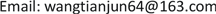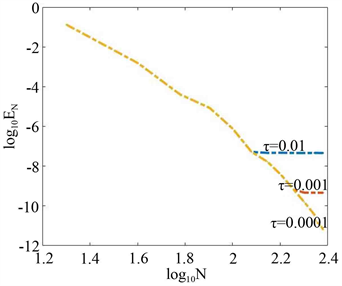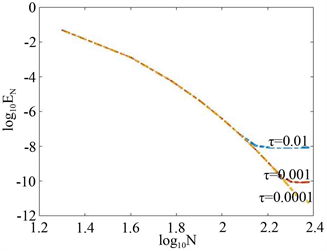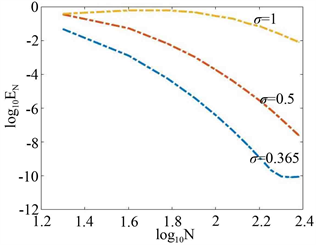﻿ Kortewego-de Vries方程的Hermite函数谱配置方法 Generalized Hermite Function Spectral-Collocation Method for KdV Equations

Vol. 08  No. 04 ( 2019 ), Article ID: 29798 , 7 pages
10.12677/AAM.2019.84070

Generalized Hermite Function Spectral-Collocation Method for KdV Equations

Bingbing Li, Tianjun Wang

College of Mathematics & Statistics, Henan University of Science & Technology, Luoyang HenanReceived: Mar. 31st, 2019; accepted: Apr. 15th, 2019; published: Apr. 22nd, 2019ABSTRACT

The paper deals with the numerical solutions of initial value problem of KdV equation on unbounded interval that approximates the solution of the Kortewego-de Vries equation, in which Hermite-Gauss nodes are used to collocate nodes of spectral collocation method. Selecting appropriately the relaxation factor $\sigma$ involved in the generalized Hermite functions approximation enables us to fit the asymptotic behaviors of exact solutions at infinity closely. Numerical results demonstrate its efficiency and high accuracy of this approach. Especially, it is much easier to deal with nonlinear equation.

Keywords:Kortewego-de Vries Equation, Initial Value Problem, Spectral Collocation Method of Hermite Functions, Hermite-Gauss Nodes

Kortewego-de Vries方程的Hermite函数谱配置方法1. 引言

$R=\left\{x|-\infty $t>0,u\left(x,t\right)$ 是实值函数。为方便，记 $\frac{\partial }{\partial x}$${\partial }_{x}$ 。考虑Kortewego-de Vries方程初值问题   

$\left\{\begin{array}{ccc}{\partial }_{t}u+\gamma u{\partial }_{x}u+\nu {\partial }_{x}^{3}u-\mu {\partial }_{x}^{5}u=0,& x\in R,& t>0,\\ u\left(x,t\right)\to 0,& |x|\to \infty ,& t>0,\\ u\left(x,0\right)=\phi \left(x\right)& x\in R,& \end{array}$ (1)

2. Lagrange插值函数及其微分矩阵

Hermite函数 ${\stackrel{^}{H}}_{n}\left(y\right)$ 定义为

${\stackrel{^}{H}}_{n}\left(y\right)=\frac{1}{\sqrt{{2}^{n}n!}}{e}^{-{y}^{2}/2}{H}_{n}\left(y\right),n\ge 0,y\in R.$

${\varphi }_{j}\left(y\right)=\frac{{e}^{-{y}^{2}/2}}{{e}^{-{y}_{j}^{2}/2}}\frac{{H}_{N+1}\left(y\right)}{\left(y-{y}_{j}\right){H}_{{}_{N+1}}^{\text{'}}\left({y}_{j}\right)},j=0,1,\cdots ,N,$

${p}_{N}^{\left(m\right)}\left(y\right)=\underset{j=0}{\overset{N}{\sum }}u\left({y}_{j}\right){\varphi }_{j}^{\left(m\right)}\left(y\right).$

${D}^{\left(m\right)}={\left({\varphi }_{j}^{\left(m\right)}\left({y}_{k}\right)\right)}_{0\le k,j\le N},D:={D}^{\left(1\right)},$

${\varphi }_{j}^{\left(1\right)}\left({y}_{k}\right)=\left\{\begin{array}{cc}\frac{{\stackrel{^}{H}}_{N}\left({y}_{k}\right)}{\left({y}_{k}-{y}_{j}\right){\stackrel{^}{H}}_{N}\left({y}_{j}\right)},& k\ne j,\\ 0,& k=j.\end{array}$ (2)

${D}^{\left(m\right)}={D}^{m}$ (3)

${H}_{n}^{\sigma }\left(x\right)={\stackrel{^}{H}}_{n}\left(\sigma x\right).$

${\varphi }_{j}^{\sigma }\left(x\right)=\frac{{\text{e}}^{-{\left(\sigma x\right)}^{2}/2}}{{\text{e}}^{-{\left(\sigma {x}_{j}\right)}^{2}/2}}\frac{{H}_{N+1}\left(\sigma x\right)}{\left(x-{x}_{j}\right){H}_{{}_{N+1}}^{\text{'}}\left(\sigma {x}_{j}\right)},j=0,1,\cdots ,N.$

${D}_{\sigma }^{\left(m\right)}={\left({\left({\varphi }_{j}^{\sigma }\left({y}_{k}\right)\right)}^{\left(m\right)}\right)}_{0\le k,j\le N},{D}_{\sigma }:={D}_{\sigma }^{\left(1\right)},$

${\left({\varphi }_{j}^{\sigma }\left({x}_{k}\right)\right)}^{\left(1\right)}=\left\{\begin{array}{cc}\frac{{H}_{N}^{\sigma }\left({x}_{k}\right)}{\left({x}_{k}-{x}_{j}\right){H}_{N}^{\sigma }\left({x}_{j}\right)},& k\ne j,\\ 0,& k=j.\end{array}$

${\varphi }_{j}^{\sigma }\left(x\right)={\varphi }_{j}\left(y\right)|{}_{y=\sigma x}$ 和(3)式知 $m$ 阶微分矩阵：

${D}_{\sigma }^{\left(m\right)}={D}_{\sigma }^{m}={\sigma }^{m}{D}^{m}$

3. Kortewego-de Vries方程的Hermite函数谱配置方法

3.1. 三阶Kortewego-de Vries方程配置方法

$\left\{\begin{array}{ccc}{\partial }_{t}u+u{\partial }_{x}u+{\partial }_{x}^{3}u=0,& x\in R,& t>0,\\ u\left(x,t\right)\to 0,& |x|\to \infty ,& t>0,\\ u\left(x,0\right)=\phi \left(x\right)& x\in R,& \end{array}$ (4)

${p}_{N}^{\sigma }\left(x,t\right)=\underset{j=0}{\overset{N}{\sum }}u\left({x}_{j},t\right){\varphi }_{j}^{\sigma }\left(x\right),x\in R$ ，逼近式(4)的解 $u\left(x,t\right)$ ，将其代入式(4)，得

$\left\{\begin{array}{cc}{\partial }_{t}{p}_{N}^{\sigma }\left({x}_{k},t\right)+{p}_{N}^{\sigma }\left({x}_{k},t\right){\partial }_{x}{p}_{N}^{\sigma }\left({x}_{k},t\right)+{\partial }_{x}^{3}{p}_{N}^{\sigma }\left({x}_{k},t\right)=0,& k=0,1,\cdots ,N,t>0,\\ {p}_{N}^{\sigma }\left({x}_{k},0\right)=\phi \left({x}_{k}\right),& k=0,1,\cdots ,N.\end{array}$ (5)

$\left\{\begin{array}{l}{u}^{\prime }\left({x}_{0},t\right)=-\underset{j=0}{\overset{N}{\sum }}u\left({x}_{j},t\right){\left({\varphi }_{j}^{\sigma }\left({x}_{0}\right)\right)}^{\left(3\right)}-u\left({x}_{0},t\right)\underset{j=0}{\overset{N}{\sum }}u\left({x}_{j},t\right){\left({\varphi }_{j}^{\sigma }\left({x}_{0}\right)\right)}^{\left(1\right)},\\ \begin{array}{cccc}& & & \begin{array}{cccc}\begin{array}{ccc}\begin{array}{cccc}\cdots & & \cdots & \end{array}& \cdots & \end{array}& & & \end{array}\end{array}\\ {u}^{\prime }\left({x}_{N},t\right)=-\underset{j=0}{\overset{N}{\sum }}u\left({x}_{j},t\right){\left({\varphi }_{j}^{\sigma }\left({x}_{N}\right)\right)}^{\left(3\right)}-u\left({x}_{N},t\right)\underset{j=0}{\overset{N}{\sum }}u\left({x}_{j},t\right){\left({\varphi }_{j}^{\sigma }\left({x}_{N}\right)\right)}^{\left(1\right)},\\ u\left({x}_{j},0\right)=\phi \left({x}_{j}\right)\begin{array}{cc},& j=0,1,\cdots N.\end{array}\end{array}$ (6)

$X\left(t\right)={\left(u\left({x}_{0},t\right),u\left({x}_{1},t\right),\cdots ,u\left({x}_{N},t\right)\right)}^{T},{X}_{0}={\left(\phi \left({x}_{0}\right),\phi \left({x}_{1}\right),\cdots ,\phi \left({x}_{N}\right)\right)}^{T}$ ，式(6)的矩阵形式为

$\left\{\begin{array}{cc}\frac{\text{d}X\left(t\right)}{\text{d}t}=-{\sigma }^{3}{D}^{3}X\left(t\right)-\sigma A\left(t\right)DX\left(t\right)& t>0\\ X\left(0\right)={X}_{0}.& \end{array}$ (7)

3.2. 数值结果

$\left\{\begin{array}{c}\left(I+\frac{1}{2}\tau {\sigma }^{3}D\right)X\left(t+\tau \right)=\left(I-\frac{1}{2}\tau {\sigma }^{3}{D}^{3}\right)X\left(t\right)-\frac{1}{2}\tau \sigma \left(A\left(t+\tau \right)DX\left(t+\tau \right)+A\left(t\right)DX\left(t\right)\right),\\ t=0,\tau ,2\tau ,\cdots T-\tau ,\\ X\left(0\right)={X}_{0}.\end{array}$ (8)

$\left\{\begin{array}{c}\left(I+\frac{1}{2}\tau {\sigma }^{3}D\right){X}_{n+1}\left(t+\tau \right)=\left(I-\frac{1}{2}\tau {\sigma }^{3}{D}^{3}\right)X\left(t\right)-\frac{1}{2}\tau \sigma \left(A\left(t+\tau \right)D{X}_{n}\left(t+\tau \right)+A\left(t\right)DX\left(t\right)\right),\\ t=0,\tau ,2\tau ,\cdots T-\tau ,\\ X\left(0\right)={X}_{0}.\end{array}$ (9)

$u=12\kappa {\text{sech}}^{2}\left(\kappa \left(x-4{\kappa }^{2}t-{x}_{0}\right)\right),$ (10)

${L}^{\infty }$ -范数

${E}_{N}=\underset{0\le j\le N}{\mathrm{max}}|u\left({x}_{j},t\right)-{P}_{N}^{\sigma }\left({x}_{j},t\right)|$Figure 1. $\kappa =0.3,\sigma =0.3$Figure 2. $\kappa =0.3,\sigma =0.3,\tau =0.001$

3.3. 五阶Kortewego-de Vries方程配置方法

${p}_{N}^{\sigma }\left(x,t\right)=\underset{m=0}{\overset{N}{\sum }}u\left({x}_{j},t\right){\varphi }_{j}^{\sigma }\left(x\right),x\in R$ ，逼近式(1)的解，将其代入式(1)，得

$\left\{\begin{array}{cc}{\partial }_{t}{p}_{N}^{\sigma }\left({x}_{k},t\right)+\gamma {p}_{N}^{\sigma }\left({x}_{k},t\right){\partial }_{x}{p}_{N}^{\sigma }\left({x}_{k},t\right)+\nu {\partial }_{x}^{3}{p}_{N}^{\sigma }\left({x}_{k},t\right)-\mu {\partial }_{x}^{5}{p}_{N}^{\sigma }\left({x}_{k},t\right)=0,& k=0,1,\cdots ,N,t>0,\\ {p}_{N}^{\sigma }\left({x}_{k},0\right)=\phi \left({x}_{k}\right)& k=0,1,\cdots ,N.\end{array}$ (11)

$\left\{\begin{array}{cc}\frac{\text{d}X\left(t\right)}{\text{d}t}=\left(\mu {\sigma }^{5}{D}^{5}-\nu {\sigma }^{3}{D}^{3}\right)X\left(t\right)-\gamma \sigma A\left(t\right)DX\left(t\right),& t>0\\ X\left(0\right)={X}_{0}.& \end{array}$ (12)

4. 数值结果

$\left\{\begin{array}{c}\left(I-\frac{1}{2}\tau \left(\mu {\sigma }^{5}{D}^{5}-\nu {\sigma }^{3}{D}^{3}\right)\right)X\left(t+\tau \right)=\left(I+\frac{1}{2}\tau \left(\mu {\sigma }^{5}{D}^{5}-\nu {\sigma }^{3}{D}^{3}\right)\right)X\left(t\right)\\ -\frac{1}{2}\tau \gamma \sigma \left(A\left(t+\tau \right)DX\left(t+\tau \right)+A\left(t\right)DX\left(t\right)\right),\\ t=0,\tau ,2\tau ,\cdots T-\tau ,\\ X\left(0\right)={X}_{0}.\end{array}$ (13)

$\left\{\begin{array}{c}\left(I-\frac{1}{2}\tau \left(\mu {\sigma }^{5}{D}^{5}-\nu {\sigma }^{3}{D}^{3}\right)\right){X}_{n+1}\left(t+\tau \right)=\left(I+\frac{1}{2}\tau \left(\mu {\sigma }^{5}{D}^{5}-\nu {\sigma }^{3}{D}^{3}\right)\right)X\left(t\right)\\ -\frac{1}{2}\tau \gamma \sigma \left(A\left(t+\tau \right)D{X}_{n}\left(t+\tau \right)+A\left(t\right)DX\left(t\right)\right)\\ t=0,\tau ,2\tau ,\cdots T-\tau ,\\ X\left(0\right)={X}_{0}.\end{array}$ (14)

$u\left(x,t\right)={\eta }_{0}+A{\text{sech}}^{4}\left(\kappa \left(x-ct-{x}_{0}\right)\right),$

$A=\frac{105{\nu }^{2}}{169\mu \gamma },\kappa =\sqrt{\frac{\nu }{52\mu }},c=\gamma {\eta }_{0}+\frac{36{\nu }^{2}}{169\mu }.$

$u\left(x,t\right)$ 作为测试函数，用 ${E}_{N}$ 度量数值误差。图3给出 $t=1$ 和不同的 $\tau$${\mathrm{log}}_{10}{E}_{N}$${\mathrm{log}}_{10}N$ 的变化关系。可以看出，数值误差随 $N$ 的增加及数值误差 $\tau$ 的减小而快速衰减，空间方向达到谱精度；图4给出 $t=1$ 和不同的伸缩因子 $\sigma$ 数值误差 ${\mathrm{log}}_{10}{E}_{N}$${\mathrm{log}}_{10}N$ 的变化关系，可以发现对适当小的 $\sigma$ 数值误差更小。但如何选取最佳伸缩因子是一个未解决的问题。Figure 3. $\sigma =0.365,\mu =\gamma =1,\nu =1.1,{\eta }_{0}=0$Figure 4. $\tau =0.001,\mu =\gamma =1,\nu =1.1,{\eta }_{0}=0$

5. 结论

Generalized Hermite Function Spectral-Collocation Method for KdV Equations[J]. 应用数学进展, 2019, 08(04): 631-637. https://doi.org/10.12677/AAM.2019.84070

1. 1. Korteweg, D. and de Vries, G. (1895) On the Change of Form of Long Waves Advancing in a Rectangular Canal, and on a New Type of Long Stationary Waves. The London, Edinburgh, and Dublin Philosophical Magazine and Journal of Science, 39, 422-443. https://doi.org/10.1080/14786449508620739

2. 2. Kawahara, R. (1972) Oscillatory Solitary Waves in Dispersive Media. Journal of the Physical Society of Japan, 33, 260-264. https://doi.org/10.1143/JPSJ.33.260

3. 3. Kichenassamy, S. and Olver, P. (1992) Existence and Nonexistence of Solitary Wave Solutions to Higher-Order Model Evolution Equations. SIAM Journal on Mathematical Analysis, 23, 1141-1166. https://doi.org/10.1137/0523064

4. 4. Wang, Z.Q. and Guo, B.Y. (2012) Legendre-Gauaa-Radau Collocation Method for Solving Initial Value Problems of First Order Ordinary Differential Equations. Journal of Sci-entific Computing, 52, 226-255. https://doi.org/10.1007/s10915-011-9538-7

5. 5. Wang, T.J. 一类线性奇异边值问题的谱配置方法[J]. 河南科技大学学报(自然科学版), 2013, 34(6): 75-78.

6. 6. Shen, J., Tang, T. and Wang, L.L. (2011) Spectral Mathod: Algo-rithms, Analysis and Applications. Springer Verlag, Berlin. https://doi.org/10.1007/978-3-540-71041-7

7. 7. 贾红丽, 王中庆. KdV方程的Chebyshev-Hermite谱配置法[J]. 应用数学与计算数学学报, 2013, 27(1): 1-8.

8. 8. 王天军. 半无界非线性热传导方程的Laguerre拟谱方法[J]. 应用数学与计算数学学报, 2013, 27(1): 9-15.

9. 9. 王天军, 杨森. 非线性Fokker-Planck方程的Hermite谱配置方法[J]. 安徽工业大学学报, 2012, 29(4): 381-384.

10. 10. Jie, S. and Tao, T. (2006) Spectral and High-Order Methods with Applications. Science Press, Beijing.

11. 11. Parkes, E., Zhu, Z., Duffy, B. and Huang, H. (1998) Sech-Polynomial Travelling Solitary-Wave Solutions of Odd-Order Generalized KdV Equations. Physics Letters A, 248, 219-224. https://doi.org/10.1016/S0375-9601(98)00662-8